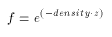# glFog

### NAME

glFogf, glFogi, glFogfv, glFogiv -- specify fog parameters

### C SPECIFICATION

void glFogf(GLenum pname,
GLfloat param)
void glFogi(GLenum pname,
GLint param)



### PARAMETERS

pname
Specifies a single-valued fog parameter. GL_FOG_MODE, GL_FOG_DENSITY, GL_FOG_START, GL_FOG_END, and GL_FOG_INDEX are accepted.

param
Specifies the value that pname will be set to.

### C SPECIFICATION

void glFogfv(GLenum pname,
const GLfloat *params)
void glFogiv(GLenum pname,
const GLint *params)



### PARAMETERS

pname
Specifies a fog parameter. GL_FOG_MODE, GL_FOG_DENSITY, GL_FOG_START, GL_FOG_END, GL_FOG_INDEX, and GL_FOG_COLOR are accepted.

param
Specifies the value or values to be assigned to pname. GL_FOG_COLOR requires an array of four values. All other parameters accept an array containing only a single value.

### DESCRIPTION

Fog is enabled and disabled with glEnable and glDisableusing the argument GL_FOG. While enabled, fog affects rasterized geometry, bitmaps, and pixel blocks, but not buffer clear operations.

glFog assigns the value or values in params to the fog parameter specified by pname. The accepted values for pname are as follows:

GL_FOG_MODE
params is a single integer or floating-point value that specifies the equation to be used to compute the fog blend factor, f. Three symbolic constants are accepted: GL_LINEAR, GL_EXP, and GL_EXP2. The equations corresponding to these symbolic constants are defined below. The default fog mode is GL_EXP.

GL_FOG_DENSITY
params is a single integer or floating-point value that specifies density, the fog density used in both exponential fog equations. Only nonnegative densities are accepted. The default fog density is 1.0.

GL_FOG_START
params is a single integer or floating-point value that specifies start, the near distance used in the linear fog equation. The default near distance is 0.0.

GL_FOG_END
params is a single integer or floating-point value that specifies end, the far distance used in the linear fog equation. The default far distance is 1.0.

GL_FOG_INDEX
params is a single integer or floating-point value that specifies if, the fog color index. The default fog index is 0.0.

GL_FOG_COLOR
params contains four integer or floating-point values that specify Cf, the fog color. Integer values are mapped linearly such that the most positive representable value maps to 1.0, and the most negative representable value maps to -1.0. Floating-point values are mapped directly. After conversion, all color components are clamped to the range [0, 1]. The default fog color is (0,0,0,0).
Fog blends a fog color with each rasterized pixel fragment's posttexturing color using a blending factor f. Factor f is computed in one of three ways, depending on the fog mode. Let z be the distance in eye coordinates from the origin to the fragment being fogged. The equation for GL_LINEAR fog isThe equation for GL_EXP fog isThe equation for GL_EXP2 fog isRegardless of the fog mode, f is clamped to the range [0 ,1] after it is computed. Then, if the GL is in RGBA color mode, the fragment's color Cr is replaced by

Cr' = f*Cr+(1-f)*Cf

In color index mode, the fragment's color index ir is replaced by

ir' = f*ir+(1-f)*if

### ERRORS

GL_INVALID_ENUM is generated if pname is not an accepted value.

GL_INVALID_OPERATION is generated if glFog is called between a call to glBegin and the corresponding call to glEnd.

### ASSOCIATED GETS

glIsEnabled with argument GL_FOG
glGet with argument GL_FOG_COLOR
glGet with argument GL_FOG_INDEX
glGet with argument GL_FOG_DENSITY
glGet with argument GL_FOG_START
glGet with argument GL_FOG_END
glGet with argument GL_MODE